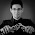## martes, 21 de noviembre de 2017

### dplyr and the design effect in survey samples

Blogdown entry here.

For those guys like me who are not such R geeks, this trick could be of interest. The package dplyr can be very useful when it comes to data manipulation and you can extract valuable information from a data frame. For example, when using if you want to count how many humans have a particular hair color, you can run the following piece of code:

library(dplyr)

starwars %>% filter(species == "Human") %>%
group_by(hair_color) %>%
summarise(n = n())
hair_colorn
auburn 1
auburn, grey 1
auburn, white 1
black 8
blond 3
brown 14
brown, grey 1
grey 1
none 3
white 2

As a result the former query gives you a data frame and you can use it to make another query. For example, if you want to know the average number of individuals in the data frame you can use the summarise twice:

library(dplyr)

starwars %>% filter(species == "Human") %>%
group_by(hair_color) %>%
summarise(n = n()) %>%
summarise(x.b = mean(n))
x.b
3.5

Now, turning our attention to statistics, it is known that, when dealing with sample surveys, one measure of interest is the design effect defined as

$Deff \approx 1 + (\bar{m} - 1)\rho$

where $\bar{m}$ is the average cluster size and $\rho$ is the intraclass correlation coefficient. If you are dealing with survey data and you want to figure out the value of $\bar{m}$ and $\rho$, you can use dplyr. Let’s use the Lucy data of the samplesize4surveys package to show how you can do it.

library(samplesize4surveys)
data(Lucy)

m <- Lucy %>% group_by(Zone) %>%
summarise(n = n()) %>%
summarise(m = mean(n))

rho <- ICC(y = Lucy$Taxes, cl = Lucy$Zone)\$ICC

DEFF <- 1 + (as.integer(m) - 1) * rho
DEFF


#### 1 comentario:

1.FYI, "count" is a shortcut for the group_by + summarise. Try this:

starwars %>% filter(species == "Human") %>%
count(hair_color)